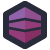As we wind up for the new year, it's time to take stock and look back at some of our best articles for the year. We start with this horrid bit of code, which hopefully has devoured itself since we posted it. --Remy

Starting with the film Saw, in 2004, the “torture porn” genre started to seep into the horror market. Very quickly, filmmakers in that genre learned that they could abandon plot, tension, and common sense, so long as they produced the most disgusting concepts they could think of. The game of one-downsmanship arguably reached its nadir with the conclusion of The Human Centipede trilogy. Yes, they made three of those movies.

This aside into film critique is because Greg found the case of a “JavaScript Centipede”: the refuse from one block of code becomes the input to the next block.

function dynamicallyLoad(win, signature) {
for (var i = 0; i < this.addList.length; i++) {
if (window[this.addList[i].object] != null)
continue;
var object = win[this.addList[i].object];
if (this.addList[i].type == 'function' || typeof (object) == 'function') {
var o = String(object);
var body = o.substring(o.indexOf('{') + 1, o.lastIndexOf('}'))
.replace(/\\/g, "\\\\").replace(/\r/g, "\\n")
.replace(/\n/g, "\\n").replace(/'/g, "\\'");
var params = o.substring(o.indexOf('(') + 1, o.indexOf(')'))
.replace(/,/g, "','");
if (params != "")
params += "','";
"=new Function('" + String(params + body) + "')");
var c = window[this.addList[i].object];
if (this.addList[i].type == 'class') {
for (var j in object.prototype) {
var o = String(object.prototype[j]);
var body = o.substring(o.indexOf('{') + 1, o.lastIndexOf('}'))
.replace(/\\/g, "\\\\").replace(/\r/g, "\\n")
.replace(/\n/g, "\\n").replace(/'/g, "\\'");
var params = o.substring(o.indexOf('(') + 1, o.indexOf(')'))
.replace(/,/g, "','");
if (params != "")
params += "','";
window.eval(String(this.addList[i].object) + ".prototype." + j +
"=new Function('" + String(params + body) + "')");
}
if (object.statics) {
window[this.addList[i].object].statics = new Object();
for (var j in object.statics) {
var obj = object.statics[j];
if (typeof (obj) == 'function') {
var o = String(obj);
var body = o.substring(o.indexOf('{') + 1, o.lastIndexOf('}'))
.replace(/\\/g, "\\\\").replace(/\r/g, "\\n")
.replace(/\n/g, "\\n").replace(/'/g, "\\'");
var params = o.substring(o.indexOf('(') + 1, o.indexOf(')'))
.replace(/,/g, "','");
if (params != "")
params += "','";
window.eval(String(this.addList[i].object) + ".statics." +
j + "=new Function('" + String(params + body) + "')");
} else
}
}
}
} else if (this.addList[i].type == 'image') {
window[this.addList[i].object] = new Image();[Advertisement] Otter - Provision your servers automatically without ever needing to log-in to a command prompt. Get started today!